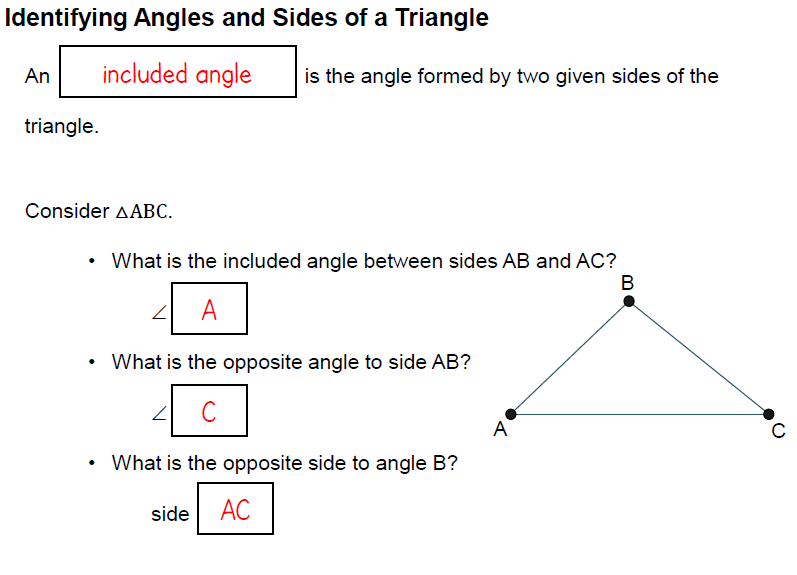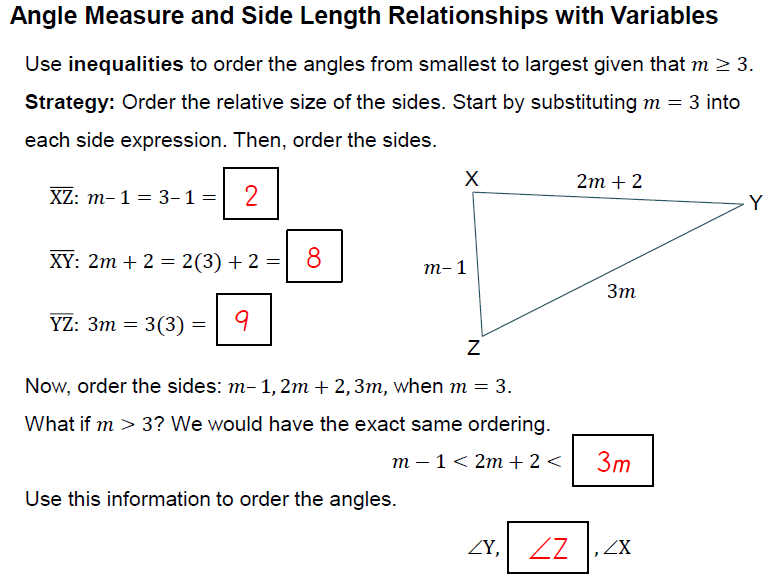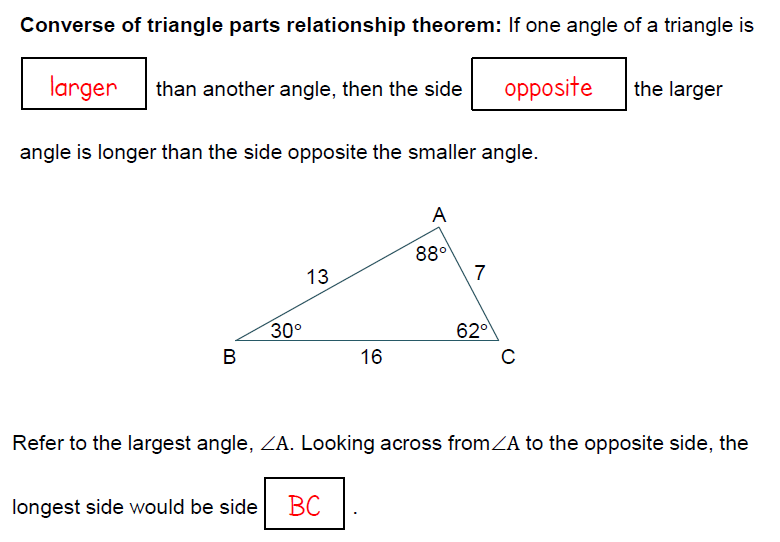# UNIT 4 LESSON 1: TRIANGLE INEQUALITIESGiven ΔGHJ, complete the following statements. The included angle of sides JG and GH is angle ________

Select all that apply
• A
• B
• C

Angle H is the opposite angle to side _______.

Select all that apply
• A
• B
• C

The side opposite to ∠J is side _____.

Select all that apply
• A
• B
• C
• D

## ANGLE MEASURE AND SIDE LENGTH RELATIONSHIPS

1. When is the largest angle, which side is the longest?

Select all that apply
• A
• B
• C

2. When is the smallest angle, which side is the shortest?

Select all that apply
• A
• B
• C

3. When side c is the longest, which angle is the biggest?

Select all that apply
• A
• B
• C

4. What is the relationship between between the angles and the side lengths for a triangle?

## PRACTICEWhat is the order of the angles from largest to smallest?

Select all that apply
• A
• B
• C
• D

## EXAMPLETriangle ABC is shown in the diagram. The lengths of the sides are in terms of the variable n, where n ≥ 4. Choose the correct inequality:

Select all that apply
• A
• B
• C
• D
• E
• F

## CONVERSE OF THE TRIANGLE PARTS RELATIONSHIP THEOREM## REAL WORLD CONNECTION

Katy and Jacque are hiking on two different trails. They reach the end of their respective trails with the straight line distance between the two trails being 5 miles. The angle between the line connecting Jacque and Katy and the line connecting Katy and the car is 50°. The angle between the line connecting Jacque and Katy and the line connecting Jacque and the car is 70°. Who has a longer distance to hike back to the car? USE THE APPLET BELOW TO HELP YOU FIND YOUR ANSWER.

## REAL WORLD CONNECTION

1. The converse of the triangle parts theorem tells us that the side that's opposite of the largest angle will be the _________ side 2. Therefore, ______ has the longer distance to hike back to the car.

Michael and Jacob are standing on two docks that are 20 miles apart. They are watching a ship that is out in the ocean. The angle between the dock and the line connecting Michael and the ship is 48°, and the angle between the other dock and the line connecting Jacob and the ship is 37°.If the ship can travel to either dock, which dock would be closest? Explain your answer.

Select all that apply
• A
• B
• C
• D